# What is Amplitude Modulation (AM)

## Definition:-

Amplitude Modulation, is a system, where the maximum amplitude of the carrier wave varies, according to the instantaneous value (amplitude) of the modulating (message or baseband) signal.

Note:-
In case of Frequency Modulation (FM) or Phase Modulation (PM), the frequency or phase respectively, of the carrier wave varies, according to the instantaneous value of the modulating (message) signal.

# FREQUENCY SPECTRUM OF AMPLITUDE MODULATION VIDEO [HD]

In the previous post, I discussed; what is amplitude modulation and its various properties. We talked about only the time domain analysis of the amplitude modulation. We saw here, how the waveform changes with time but here, we will discuss the frequency domain analysis of the amplitude modulation. Here you will see, how the spectrum of modulating signal, carrier signal and modulated wave looks like. I will also derive here the equation of AM wave in frequency domain. So let's start...

# Amplitude Modulation (Time Domain Equations)

Now look at the Image given below, that is self explanatory (Click the Image to enlarge)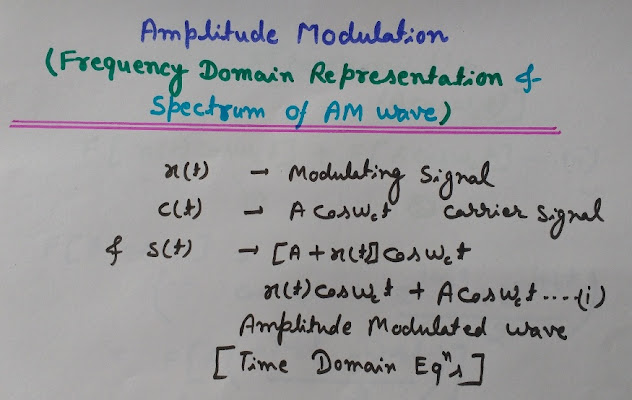Amplitude Modulation Equations in time domainImage (1)

As per the discussion in previous post on Amplitude Modulation, we know that-

x(t) Modulating signal (also called as Message signal or Baseband signal)
c(t) = Acos(wct)   Carrier wave
s(t) = [A+x(t)]cos(wct)
=x(t) cos(wct)+ Acos(wct) Amplitude Modulated wave…(i)

Here ‘A’ is the amplitude of the carrier wave and
‘wc’ is the angular frequency of the carrier wave

## Fourier Transform of Waves (Spectrum i.e. Frequency Domain Representation of the Waves)

Here all the equations are in time domain, so to get the spectrum of all these waves, i.e. frequency domain representation of the waves, we need to find out the Fourier transform (FT) of the waves.
The Fourier Transform (FT) will convert the Time Domain into Frequency Domain.

Now see the Image given below carefully (Click the image to enlarge)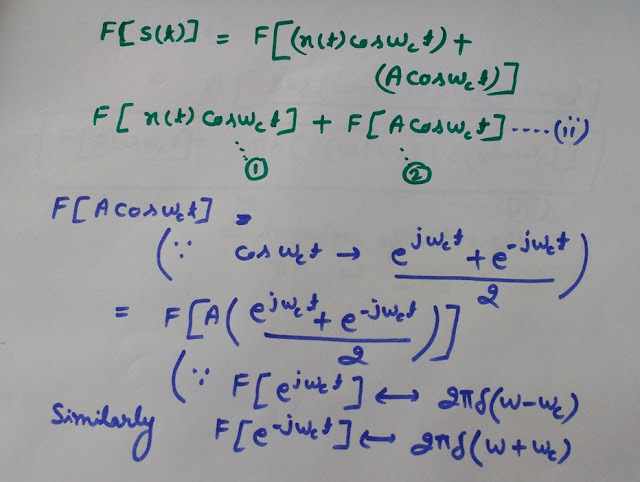Calculation of Fourier Transform of Amplitude Modulated Wave Image (2)

Here as per the image-
We are now going to find the Fourier Transform (FT) of the Amplitude Modulated Wave, s(t).

F[s(t)]= F[x(t) cos(wct) + Acos(wct)]
= F[x(t) cos(wct)] + F[Acos(wct)]…(ii)

The equation (ii), has two parts, Lets say..
x(t) cos(wct)     Part 1 &
Acos(wct)         Part 2
So let's find out the Fourier Transform of each of these parts separately.
First,we will start with finding the Fourier transform of the part 2 of equation (ii).

On doing all the calculations, we derived the Fourier Transform (FT) of Part 1 of AM Wave, that is given below and also in the image as equation (iii)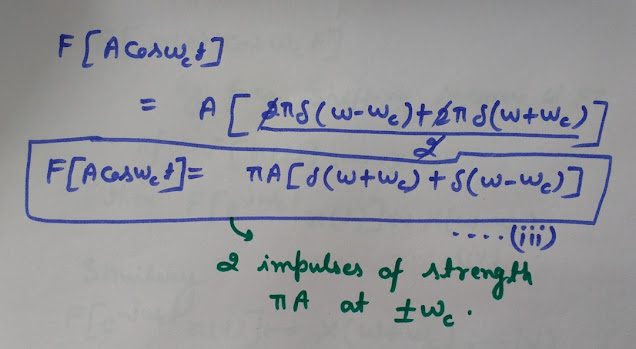Calculation of Fourier Transform of Amplitude Modulated Wave Image (3)

FT of [Acos(wct)]= Pi A[Delta(w+wc)+Delta(w-wc)]…(iii)

We will need this later, after finding the FT of another part of the Amplitude Modulated Wave.
So one important thing that you should notice here is that, this equation (iii) means, Fourier transform of “Acos(wct)” has two impulses of strength (Amplitude) "Pi A” at “+- Wc”.
We will see this, when we will draw the spectrum of the waves, Now let’s calculate the Fourier transform of another part.

Now you need to see the Image given below-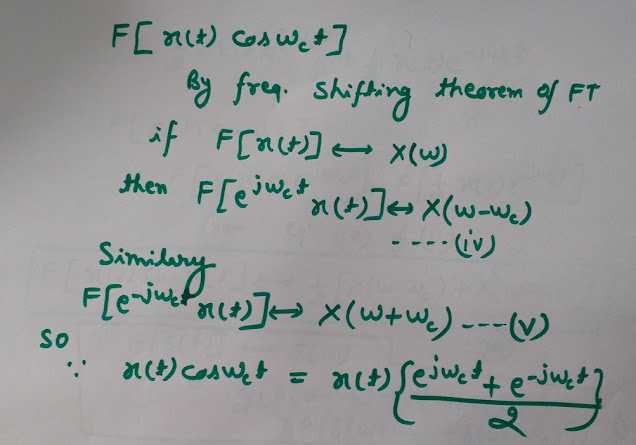Calculation of Fourier Transform of Amplitude Modulated Wave Image (4)

According to the image-
By frequency shifting theorem of Fourier transform we know that, if the Fourier transform of x(t) is X(w) then the Fourier transform of [e^(jwct)x(t)], i.e. if we multiply x(t) signal with [e^(jwct) then its Fourier transform would be X(w-wc), let’s call this as equation (iv).
In the same way we can write
FT of [e^(-jwct)x(t)] is X(w+wc)…(v)
So from equations (iv) and (v),
The FT of x(t)[cos(wct)] is, (See the derivation in Image 4 and Image 5)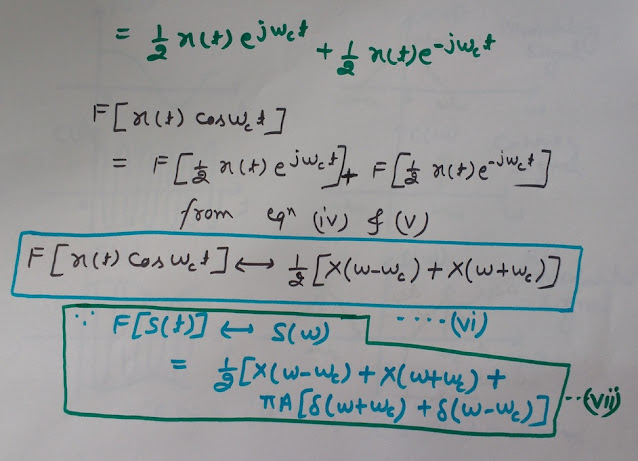Calculation of Fourier Transform of Amplitude Modulated Wave Image (5)

F[x(t){cos(wct)}] =1/2[X(w-wc)+X(w+wc)]…(vi)

This equation (vi) means, that on multiplying x(t) by
cos(wct), the spectrum of X(w) shifts by (+-wc).
Now we will combine, the Fourier Transforms of both parts of the Amplitude modulated wave, Part 1 and Part 2 of equation (ii),
that we calculated in the equations (iii) and (vi).
So the final equation of Amplitude modulated wave in Frequency Domain (Fourier Transform of the AM wave)

If F[s(t)] is S(w) then
S(w) = 1/2[X(w-wc)+X(w+wc)] + Pi A[Delta(w+wc)+Delta(w-wc)]

You can see this equation in the image below-Final Equation of Amplitude modulated wave  in Frequency Domain (Fourier Transform) Image (6)

## Waveform (Time Domain) and Spectrum (Frequency Domain) of the Amplitude Modulation

So this was the Mathematical part including equations and derivations, now we will see the waveform of these waves in time domain and in frequency domain (Spectrum).

Here I will draw the waveform (time domain) and spectrum (Frequency domain) of the modulating signal x(t), carrier signal c(t) and amplitude modulated wave s(t).

Please see the Image given below (Click on the image to enlarge) for all the waveform in time domain and also the spectrum.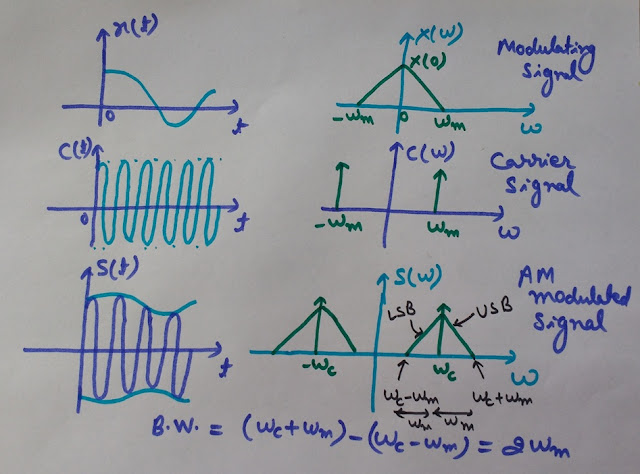Time Domain waveforms and Spectrum of AM modulation Image (7)

From the waveforms (time domain) in the image, we can see that

Modulating signal x(t) can have (as in this case)
Multiple Frequencies and
Variable Amplitude

Carrier wave c(t) (a high frequency wave used as a carrier of the message signal (modulating signal) has (in this case)
Constant amplitude
Constant frequency (High frequency)

Amplitude Modulated wave s(t) has-
Variations in Carrier wave Amplitude, that varies as per instantaneous value of the modulating signal (message signal). This varying amplitude present in the Amplitude modulated wave contains information about the message and is called as the Envelope of the wave. So the information is contained in the envelop, that can be extracted at the receiving end by the process of demodulation.
[Since it is amplitude modulation, therefore the amplitude of the carrier wave varies, according to the instantaneous value of the modulating signal. In case of Frequency modulation (FM) or phase modulation (PM), the frequency or phase respectively, of the carrier wave varies, according to the instantaneous value of the modulating signal.]
Now let’s discuss the Spectrum of these waves:-

### Spectrum of Modulating wave X(w)

It has a triangular shape having frequencies at (+-wm)
Here wm is the highest frequency present in the modulating signal.

### Spectrum of the carrier wave C(w)

It has two impulses at +wm and –wm

This thing we have seen already in the equation (iii) given below-

FT of [Acos(wct)]= Pi A[Delta(w+wc)+Delta(w-wc)]…(iii)

Fourier Transform gives the spectrum (frequency domain) of the waveform in time domain.

### Spectrum of amplitude modulated wave S(w)

It contains two identical and symmetrical triangular shapes.
These two parts are the replicas of each other.

If F[s(t)] is S(w) then
S(w) = 1/2[X(w-wc)+X(w+wc)] + Pi A[Delta(w+wc)+Delta(w-wc)]

From the image you can easily observe that each of the triangular part contains two sidebands known as Upper Side Band (USB) and Lower Side Band (LSB).
Observe the image 7 that-
wc>wm
Because of this, the two sidebands i.e. LSB and USB don’t overlap.

We can easily calculate the Bandwidth of the Amplitude modulated wave from its spectrum-
As you can see in the spectrum shown in the image-

Bandwidth = (wc+wm) -  (wc-wm)
= 2 wm (equal to the length of the base of the triangle in the spectrum)

Here wm is the maximum frequency present in the modulating
signal and wc is the Frequency of the carrier wave.

Here I want to tell you one more thing that the negative frequencies in the spectrum of the modulated wave are due to the Fourier transform of exponential signal and are just for mathematical representation. In reality these negative frequencies have no significance. But as we know that positive and negative parts in the spectrum are replica of each other, so all that we need can be calculated only by the positive side of the frequencies in the spectrum.

1.1.The effectiveness of IEEE Project Domains depends very much on the situation in which they are applied. In order to further improve IEEE Final Year Project Domains practices we need to explicitly describe and utilise our knowledge about software domains of software engineering Final Year Project Domains for CSE technologies. This paper suggests a modelling formalism for supporting systematic reuse of software engineering technologies during planning of software projects and improvement programmes in Final Year Project Centers in Chennai.

Spring Framework has already made serious inroads as an integrated technology stack for building user-facing applications. Spring Framework Corporate TRaining the authors explore the idea of using Java in Big Data platforms.
Specifically, Spring Framework provides various tasks are geared around preparing data for further analysis and visualization. Spring Training in Chennai

2.3.4.5.6.The Source of medications for recreational use was brought into the US with 'smoking' parlors opening in a few significant urban communities.

7.8.9.